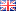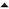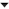## Facebook Hacker Cup 2016: Round 1 problem analysisThe first round of the Facebook Hacker Cup has just concluded! As of writing, there are over 600 competitors that have submitted solutions for all four problems. Since only the top 500 advance (including anyone tied for 500th place) a perfect score may be a requirement, though it's also possible that a significant fraction of those solutions turns out to be wrong, as the final results have not yet been announced.

edit:
The scoreboard has been updated. 395 people scored 100 points. The minimum score necessary to advance is 85 points, and that covers the top 560.

In the context of the entire contest, Round 1 is quite unique, because it's the only round where accuracy is of critical importance and solution speed does not matter (that is, as long as you manage to solve the problems within 24 hours). Last year, my accuracy was less than perfect and I failed to advance. Hopefully, I did better this year!

Fortunally, the problems were not too difficult. Let's have a look!

#### Problem A: Coding Contest Creation (10 points)

Problem statement (official link)
We're given a list of problem difficulties, and we should partition it into chunks of at most 4 problems at a time, such that the difficulties are strictly increasing, and the gaps between difficulties are at most 10. We're allowed to create new problems to fill the gaps, and should calculate the minimum number of new problems necessary.

This is another problem where a greedy approach will work: we start the first contest with the first problem from the list, and then keep adding problems from the list to the contest while we can. If the next difficulty is either too high (more than 10 points higher than the last) or too low (less than or equal to the last) then we might as well write a new problem with difficulty 10 greater than the last one, because either we'll get closer to the next problem, or we can't use it anyway.

There are many ways to implement fundamentally the same logic. My submission looked like this:
Python:
 ```1 2 3 4 5 6 7 8 9 10 11 12 13 14 15 16 17 18 19 20 ``` ```from sys import stdin def solve(N, D): assert len(D) == N D.append(0) extra = i = 0 while i < N: for n in range(4): if n == 0 or last < D[i] <= last + 10: last = D[i] i += 1 else: last += 10 extra += 1 return extra for case in range(1, int(stdin.readline()) + 1): N = int(stdin.readline()) D = map(int, stdin.readline().split()) print('Case #{}: {}'.format(case, solve(N, D)))```

To sanity-check my results, I also wrote a dynamic programming solution, which simply tries to construct a contest out of 1, 2, 3 or 4 items from the input:
Python:
 ```1 2 3 4 5 6 7 8 9 10 11 12 ``` ```def increasing(x): return all(x[i] < x[i + 1] for i in range(len(x) - 1)) def extra_needed(x): return sum((x[i + 1] - x[i] - 1)//10 for i in range(len(x) - 1)) def solve(N, D): cost = *(N + 1) for i in reversed(range(N)): cost[i] = min(cost[i + n] + (4 - n) for n in (1, 2, 3, 4) if i + n <= N and increasing(D[i:i + n]) and n + extra_needed(D[i:i + n]) <= 4) return cost```

Both versions take time proportional to the size of the input. That's as fast as you can get!

#### Problem B: Laundro, Matt (20 points)

Problem statement (official link)
The second problem is a simulation problem. These kind of problems typically don't require deep insight or theoretical knowledge; you just have to faithfully implement the process as it is described in the problem statement. However, that doesn't mean it's easy! In fact, simulation problems are often very tricky to implement, because all details must be exactly right in order to get the correct results.

In this case, the main difficulty comes from the fact that the washing and drying times can be very large (up to 1 billion minutes -- FYI: that's over 1900 years; Matt must be really patient!) Since we cannot simulate every minute in time, we have to fast-forward to the next interesting event.

Another tricky question is how to pick which washing machines to use. It seems tempting to use the fastest one that's available, but that may not be optimal: consider the case where one machine takes 1 minute, and another 10. If you have three loads, it's better to schedule them all on the first machine, than to run two loads in parallel. The general principle here, is that you should always schedule your load in the machine that would finish it the soonest.

The same dilemma does not apply to the available dryers, since they all take the same time to finish a load.

To implement the simulation, we'll maintain two priority queues, one for each type of machine. The washers are sorted by the time they could finish the next load (if you chose to schedule another one on that machine), while the dryers are just sorted by the time they'll become available. To implement these queues, I used Python's heapq standard library which implements a priority queue as a binary heap. Heaps are pretty cool, but you don't need to understand them to use the heapq functions. All you need to know is that they allow you to maintain a list in such a way that you can efficiently remove the smallest element from it.

There's one final tricky part: the number of dryers can also be very large. Because we'll never need more than L, the number of loads, we'll just initialize our list with the minimum of L and M. Finally, since every load must be washed and dried, our answer will be equal to the time the last dryer finishes.

Now all details have been addressed, the implementation almost writes itself:
Python:
 ```1 2 3 4 5 6 7 8 9 10 11 12 13 14 15 16 17 18 19 ``` ```from sys import stdin from heapq import heappush, heappop, heapify def solve(L, N, M, D, W): assert len(W) == N washers = [(w, w) for w in W] heapify(washers) dryers = *min(M, L) for _ in range(L): t, w = heappop(washers) heappush(washers, (t + w, w)) u = heappop(dryers) heappush(dryers, max(t, u) + D) return max(dryers) for case in range(1, int(stdin.readline()) + 1): L, N, M, D = map(int, stdin.readline().split()) W = map(int, stdin.readline().split()) print('Case #{}: {}'.format(case, solve(L, N, M, D, W)))```

#### Problem C: Yachtzee (25 points)

Problem statement (official link)
The third problem involves probability theory. There's a random variable (sampled uniformly from the range [A:B]) and a piecewise valuation function, and we're asked to calculate the expected value. Intuitively, the expected value is equal to the average value if you picked a random element between A and B, evaluated the answer, and then repeated this an infinite number of times. However, we can calculate the value exactly without doing any simulations. The only tricky part is, again, that all parameters can be very large (although it turned out the testdata was not as complicated as the constraints in the problem statement allowed).

Let's start with an easy case. Let's say our yacht requires one part, which costs \$10, and we have between \$0 and \$10. What is the expected value that we end up with? Since we sample uniformly between \$0 and \$10, the probability that we get exactly \$10 is 0%. And since we can get any value between \$0 and \$10 with equal probability, then the average value is \$5.

That was easy, right? Now suppose there are two parts, costing \$10 and \$20 respectively, and we have between \$0 and \$30. Now, we have 1/3rd chance that we have less than \$10, and 2/3rd chance that we have between \$10 and \$30. In the first case, we have an expected \$5 left as before. In the second case, we buy the \$10 part, and have anywhere between \$0 and \$20 left, with an average of \$10. The expected value in that case is 1/3*\$5 + 2/3*\$10 = \$8.33. A different way to write that is (10*\$5 + 20*\$10)/30: here it's clearer that we multiply each average by the size of the range, and then divide the sum by the total cost of the yacht.

Now we know how to calculate the expected amount of money left if our money ranges between \$0 and the cost of all parts (let's say: \$yacht). But what if the range is something else? In particular, what if A > \$0 and B < \$yacht? We can use the same logic. For example, in the same case above, let's say we have at least \$5 and at most \$25. That means that we have 1/4th chance to have less than \$10, but if we do, the average is \$7.50 (between \$5 and \$10). Similarly, we have 3/4th chance that we have more than \$10. In that case, we buy the \$10 part, and then we have an average of \$7.50 left (between \$0 and \$15). The weighted average will be (5*\$7.50 + 15*\$7.50)/20 = \$7.50, unsurprisingly.

Clearly we can calculate any expected value as long as our range of money [A:B] falls within the cost of the yacht [0:\$yacht]. But what if it doesn't? First, let's consider the case that A >= \$yacht. If x*\$yacht <= A < (x+1)*\$yacht, that means we will always buy a minimum of x yachts. In that case, the answer for the range [A:B] will really be the same as for [A - x*\$yacht:B - x*\$yacht]. If B <= (x+1)*yacht, that means we've reduced our range to within [0:\$yacht] and we can solve it. However, if y*\$yacht <= B < (y+1)*\$yacht for some y>x, that means there are some cases where we buy different number of yachts.

To deal with those, let's use a theorem from probability theory: since we are sampling uniformly, we can split up a range [A:B] into two parts [A:X] + [X:B] (where A <= X <= B ) and then the expected value E([A:B]) = ((X - A)*E([A:X]) + (B - X)*E([X:B]))/(B - A). That might sound complicated, but if you look closely, it's just the same weighted average that we've been using before to calculate the expected value for a single yacht. We can use this information to split up a range [A:B] into [A:(x + 1)*\$yacht] + [(x + 1)*\$yacht:(x + 2)*\$yacht] + ... + [(y - 1)*\$yacht:y*\$yacht] + [\$y*yacht:B]. All of these ranges we can solve individually and then we can calculate the combined expected value as a weighted average.

But wait! What if the number of ranges is very large? After all, B can be up to a billion, and the total price of a yacht, \$yacht, might be very small. Indeed, calculating so many little ranges might take too long. So we need a final trick: all ranges of the form [(x + k)*\$yacht:(x + k + 1)*\$yacht] are really the same, and their value is equal to that of [0:\$yacht]. So we can compute that range once, and multiply by the number of times it occurs. That means our final formula will be: [A:(x + 1)*\$yacht] + (y - x - 1)*[0:\$yacht] + [\$y*yacht:B] (where x and y, as before, are the minimum and maximum number of yachts we could buy).

Phew! That was a lot of text. The good news is that the code is comparatively simple. You just need to be careful to avoid integer overflow, and to make sure you convert integers to floating point numbers when you need to divide (i.e. to calculate a weighted average):
Python:
 ```1 2 3 4 5 6 7 8 9 10 11 12 13 14 15 16 17 18 19 20 21 22 23 24 25 26 27 28 29 30 31 32 33 ``` ```from sys import stdin def average_sum(x): return x * x / 2.0 def solve(N, A, B, C): yacht_cost = sum(C) def weight(a, b): assert 0 <= a <= b <= yacht_cost w = 0.0 for c in C: if b > 0 and a < c: w += average_sum(min(b, c)) w -= average_sum(max(a, 0)) a -= c b -= c return w min_yachts = A // yacht_cost max_yachts = B // yacht_cost if min_yachts == max_yachts: total_weight = weight(A - yacht_cost*min_yachts, B - yacht_cost*max_yachts) else: total_weight = (weight(A - yacht_cost*min_yachts, yacht_cost) + weight(0, yacht_cost)*(max_yachts - min_yachts - 1) + weight(0, B - yacht_cost*max_yachts)) return total_weight / (B - A) for case in range(1, int(stdin.readline()) + 1): N, A, B = map(int, stdin.readline().split()) C = map(int, stdin.readline().split()) print('Case #{}: {:.19f}'.format(case, solve(N, A, B, C)))```

#### Problem D: Boomerang Tournament (40 points)

Problem statement (official link)

Compared to the previous problem, the forth and final problem of Round 1 was surprisingly simple. At the core, it's just another simulation problem. However, since execution speed matters, I implemented my solution only in C++.

To start tackling the problem, the first thing to notice is that the number of competitors will be fairly small: only up to 16. It would be simple to try all permutations individually, and track the best and worst outcome for each competitor. However, there are 16! = 20,922,789,888,000 permutations (where ! denotes the factorial function), which is just a tad too high. We'll have to be a little smarter.

The second thing to notice is that the problem statement promises that the number of contestants will be a power of 2. That's convenient, because it means we can ignore the "queue" from the problem statement, and just view the contest as series of rounds: in each round, every contestant is matched up against someone else, half of the contestants are eliminated, and so on until one winner remains. The order in which players battle within a round is completely irrelevant, since the rank is based only on the number of rounds a player survives. That's also why there are only five possible ranks in the output: 1, 2, 3, 5, 9. The significance of those numbers is more obvious if we subtract 1: 0, 1, 2, 4, 8, corresponding with the number of players that advance each round.

Because we're ignoring the order of matches within a round, we don't need to consider 16! permutations but only nCr( 16, 8 ) = 12,870 combinations (that's the number of ways to pick 8 winners from a set of 16 competitors). That's a pretty small number. We can use backtracking to generate all possible subsets; we'll generate some combinations twice (since there may be multiple matchings that result in the same set of winners/losers: for example, A beats C and B beats D, is equivalent to A beats D and B beats C). To make sure we don't do any unnecessary work, we'll track which sets of winners/losers we've seen before, and process each set only once.

The code below implements this idea, using bitmasks to represent subsets of contestants. There are a few bit-twiddling tricks and built-in functions used, that may need some explaining:
• __builtin_popcount(mask) returns the number of 1-bits in mask ("popcount" stands for population count).
• __builtin_ctz(mask) returns the 0-based index of the lowest bit set. ("ctz" stands for "count trailing zeros": i.e. how many zeroes are there to the right of the last 1 bit? The answer is exactly equal to the index of that 1 bit.)
• mask & (mask - 1) evaluates to 0 if (and only if) there is only one bit set in mask.
C++:
 ```1 2 3 4 5 6 7 8 9 10 11 12 13 14 15 16 17 18 19 20 21 22 23 24 25 26 27 28 29 30 31 32 33 34 35 36 37 38 39 40 41 42 43 44 45 46 47 48 49 50 51 52 53 54 55 56 57 58 59 60 61 62 63 64 65 66 67 68 ``` ```#include #include #include #include using namespace std; static vector> W; static vector best_rank; static vector worst_rank; static vector seen_winners; static vector seen_losers; unsigned Bit(int i) { return 1u << i; } void Solve(unsigned unmatched, unsigned winners, unsigned losers) { if (unmatched) { int i = __builtin_ctz(unmatched); for (int j = i + 1; Bit(j) <= unmatched; ++j) { if (unmatched & Bit(j)) { int winner = W[i][j] ? i : j; int loser = W[i][j] ? j : i; Solve(unmatched - Bit(i) - Bit(j), winners + Bit(winner), losers + Bit(loser)); } } } else { if (!seen_losers[losers]) { int rank = __builtin_popcount(winners) + 1; for (int i = 0; Bit(i) <= losers; ++i) { if (Bit(i) & losers) { best_rank[i] = min(best_rank[i], rank); worst_rank[i] = max(worst_rank[i], rank); } } seen_losers[losers] = true; } if (!seen_winners[winners]) { seen_winners[winners] = true; if ((winners & (winners - 1)) == 0u) { best_rank[__builtin_ctz(winners)] = 1; } else { Solve(winners, 0u, 0u); } } } } int main() { int T = 0; cin >> T; for (int t = 1; t <= T; ++t) { int N; cin >> N; W.assign(N, vector(N)); for (int i = 0; i < N; ++i) for (int j = 0; j < N; ++j) cin >> W[i][j]; seen_winners.assign(Bit(N), false); seen_losers.assign(Bit(N), false); best_rank.assign(N, N/2 + 1); worst_rank.assign(N, 1); Solve(Bit(N) - 1, 0, 0); cout << "Case #" << t << ":\n"; for (int i = 0; i < N; ++i) cout << best_rank[i] << ' ' << worst_rank[i] << '\n'; } }```01-'16 Facebook Hacker Cup 2016: Round 2 problem analysis01-'16 Facebook Hacker Cup 2016: Qualification Round problem analysis